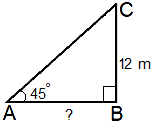# The angle of elevation of the sun is 45° then the length of the shadow of a 12 m high tree is …… A) 12√3

30 views

The angle of elevation of the sun is 45° then the length of the shadow of a 12 m high tree is ……

A) 12√3

B) 16

C) 12

D) 16 √3

+1 vote
by (34.0k points)

Correct option is: C) 12

by (12.6k points)

Correct option is: C) 12We have the height of the tree is BC = 12 m.

Angle of elevation of the sum is $\angle$A = $45^\circ$

In right $\triangle$ABC,

$\frac {BC}{AB}$ = tan A = tan $45^\circ$ = 1

= AB = BC = 12 m

Hence, the length of the shadow of the tree is 12 m.